# Part 5: Rates and Ratios

Mastering Ratio and Rates in Year 8 is a crucial gateway to being able to successfully navigate through senior mathematics and secure your fundamentals. Here is our guide to ensuring your success with some tips that you should check out before going on to Year 9.Are you confident with your Rates and Ratios skills? In this guide, we will show you how to approach different problem questions involving rates and ratios and help you investigate, interpret and analyse graphs.

## NSW Syllabus outcome

 Syllabus Explanation NSW Stage 4 NESA Syllabus Solve a range of problems involving ratios and rates, with and without the use of digital technologies (ACMNA188) Solve a variety of real-life problems involving ratiosSolve a variety of real-life problems involving rates, including problems involving rate of travel (speed) Investigate, interpret and analyse graphs from authentic data (ACMNA180) Interpret distance/time graphs (travel graphs) made up of straight-line segmentsRecognise concepts such as change of speed and direction in distance/time graphs

## Assumed knowledge for Rates and Ratios

Students should understand how to write, interpret and simplify simple ratios.

This guide aims to enrich these skills with techniques and advice that Matrix students learn.

## Applying the Unitary Method

A rate is a comparison of quantities which are measured in different units.

I.e. a rate can be thought of as:

First Unit / Second Unit

The unitary method is often used to apply rates in problems and involves writing the first unit as per ONE of the second unit.

This ‘simplified rate’ can then be then applied as required by the question.

The steps for using this method is as follows:

### Step 1: Remove any fractions or decimals

In order to simplify the rate, it is important to first work in integers.

If the rate involves decimals, multiply both parts by an appropriate power of $$10$$.

For example, the rate is given as:

\begin{align*}
0.333m /  \ 4s
\end{align*}

We identify that only the $$LHS$$ is a decimal.

To change this decimal into an integer, we must multiply by $$1000$$ (i.e. $$10^3$$).

However, it is important to remember that we must do the required operations to both sides of the ratio.

Then we will have:

\begin{align*}
333 \ m /  \ 4000s
\end{align*}

Now we are working with integers.

If we were given the rate in fractions, we would instead multiply both sides by the  LCM of the denominators.

For example:

\begin{align*}
\frac{2}{3} L \ / \ \frac{3}{8}s
\end{align*}

Here we can see that the LCM of the denominators is $$24$$.

Therefore, we multiply both sides of the fraction by $$24$$ to get:

\begin{align*}
16L \ / \ 9s
\end{align*}

Again, we are now working with integers.

### Step 2. Divide both sides by the second unit

The next step is to divide both sides by the second unit.

This will then give us the first unit per ONE of the second unit.

For example:

\begin{align*}
16L \ / \ 9s
\end{align*}

Here the second unit has a value of $$9$$.

Therefore, both sides should be divided by $$9$$:

\begin{align*}
\frac{16}{9} L \ / \ 1s
\end{align*}

### Step 3: Apply the simplified rate

The last step is to then apply this simplified rate to the question.

This will often involve multiplying both sides by a certain number in order to obtain a scaled rate which directly applies to the question.

For example:

1. How many litres of water will flow into a tank after $$32$$ seconds, given that the water flows in at a rate of $$16 L \ / \ 9 s$$?

Solution:

To solve this question, we would use our simplified rate from before:

\begin{align*}
\frac{16}{9} L \ / \ 1s
\end{align*}

To find out how much water flows in after $$32 \ seconds$$, we would need multiply both $$LHS$$ and $$RHS$$ by $$32 \ seconds$$.

\begin{align*}
\frac{512}{9}L \ / \ 32s
\end{align*}

Therefore, we can easily see that $$\frac{512}{9} L$$ of water would have flowed into the tank after $$32$$ seconds.

### Common mistakes

Common mistakes students make with travel graphs are:

• Not multiplying across both $$LHS$$ and $$RHS$$
• Incorrectly identifying the second unit.

## Real-life Problems: Speed

Speed is one of the most common rates encountered in real life.

Speed compares distance with time through the following formula:

\begin{align*}
Speed \ = \ \frac{Distance}{Time}
\end{align*}

Speed can be expressed through many different units.

However the most common ones are $$m/s$$ (metres per second) or $$km/h$$ (kilometres per hour).

The above formula can also be rearranged to obtain expression for distance and time:

\begin{align*}
Distance \ = \ Speed \times \ Time
Time \ = \ \frac{Distance}{Speed}
\end{align*}This triangle/formulae are extremely important when applying rates to solve questions involving speed.

Below are some techniques/rules on how to apply these formulas for worded questions:

### Step 1: Write down the given quantities

The first step in solving any speed/distance/time questions which involve rates is to write down the known quantities.

For example:

1. Amanda drove from Newcastle to Sydney. She left Newcastle at $$1:30 pm$$ and arrived at Sydney at $$4:30 am$$ the next day. If she drove a total distance of $$5427 \ kilometres$$, what was her average speed?

Solution:

In the question above we can clearly discern two quantities:

\begin{align*}
\text{Distance} &= 5427 km \\
\text{Time} &= \text{Time between} \ 1:30 pm \ \text{and} \ 4:30 am \ \text{the next day} \\
&= 9 \ hours
\end{align*}

### Step 2: Choose the relevant equation using the known quantities

Continuing on from the question above, we are given distance and time and we can see that we want to solve for speed.

Hence we would use the formula:

\begin{align*}
Speed \ = \ \frac{Distance}{Time}
\end{align*}

Applying the given quantities we get:

\begin{align*}
Speed \ &= \ \frac{5427 \ km }{9 \ hours} \\
&= 603 \ km \ / \ h
\end{align*}

### Common mistakes:

Common mistakes students make with travel graphs are:

• Confusing the different formulas.
• Not ensuring that all the quantities are in the same units before beginning calculations.

## See your marks rise high## Converting Rates between Different Units

Often questions will ask to convert rates between different units.

For these questions, it is important to be wary of when to divide and when to multiply the rate.

Below is a generic step-by-step guide for converting rates:

### Step 1: Determine whether the first unit, second unit or both need to be converted.

It is important to determine which part of your rates need to be converted.

For example:

a) If we are trying to convert $$9 km/h \ into \ m/s$$

We need to change both our first unit $$(km)$$ and our second unit $$(h)$$.

b) If we are trying to convert $$12 km/h \ into \ km/s$$

We need to only change the second unit $$(h)$$.

### Step 2: Systematically work through converting each unit one at a time

Always convert each unit one at a time.

The order is not consequential, but in this guide, we start by converting the second unit into the required format and then work on converting the first unit.

E.g. To convert $$9 km/h$$ into $$m/s$$

1. First convert the rate to $$x \ km/s$$
2. Then convert to $$y \ m/s$$.

Converting the second unit

When converting the second unit from larger measurements (e.g.  $$hours$$) into smaller measurements (e.g. $$seconds$$):

• You always divide by an appropriate number.

When converting the second unit from smaller measurements (e.g. $$seconds$$) into larger measurements (e.g. $$hours$$):

• You always multiply by an appropriate number.

The following diagram illustrates how to convert time, the most common second unit: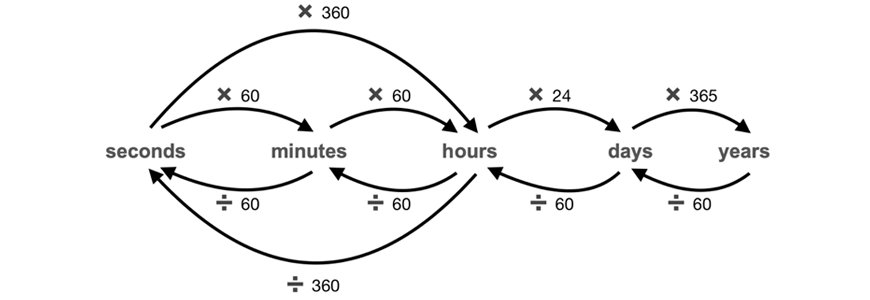Therefore, for above example, we would divide $$9$$ by $$360$$ to convert from $$9 \ km/h$$ to $$0.025 \ km/s$$.

Converting the first unit

The next step is to convert the first unit. The rules for converting the first unit are the opposite.

When converting the first unit from larger measurements (e.g. $$kilometres$$ ) into smaller measurements (e.g. $$metres$$ )

• You always multiply by an appropriate number.

Similarly, when converting the first unit from smaller measurements (e.g. $$metres$$ ) into larger measurements (e.g. $$kilometres$$ )

• You always divide by an appropriate number.

The following diagram illustrates how to convert length/distance, the most common first unit: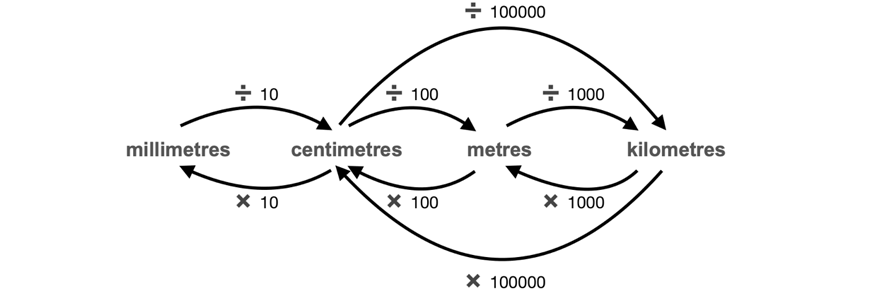Hence, continuing on from the first example…

To convert $$0.025km/s$$ into $$y \ m/s$$, we multiply $$0.025$$ by $$1000$$.

This leaves us with an answer of $$25 m/s$$.

### Example

1. Riley walks at a speed of $$10 \ 342 \ 080 \ mm/day$$. Convert this rate into $$km/s$$.

Solution:

First convert $$10 \ 342 \ 080 \ mm/day$$ into $$x \ mm/s$$.

To do this, divide by $$8640$$:

$$1197 mm/s$$

Then convert into $$y \ km/s$$.

To do this, divide by $$1 \ 000 \ 000$$:

$$0.001197 km/s$$

### Common mistakes

Common mistakes students make when converting rates are:

• Confusing the difference between changing the first unit and second unit
• Forgetting the value of key terms (i.e. how many zeros in a tonne, etc.).

## Distance-Time (Travel) Graphs

Travel Graphs are a staple of the Rates topic.

It can be used to obtain fairly in-depth information about a subject’s journey.

The $$x axis$$ always represents time whereas the $$y axis$$ always represents distance.

An example graph is shown below: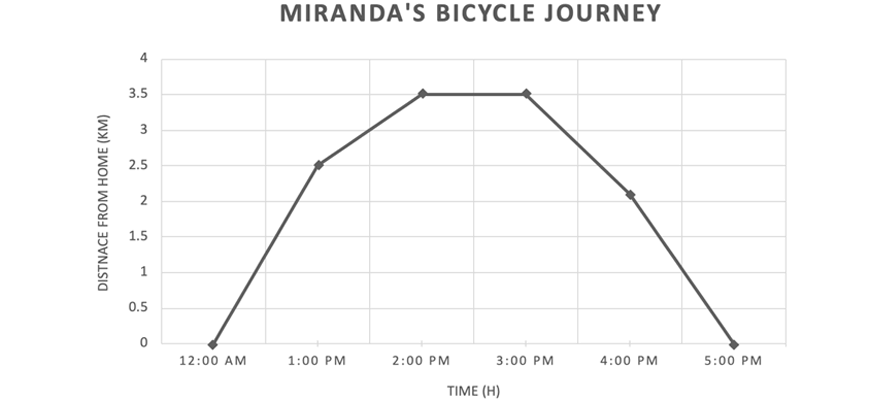From the graph above it is important to note certain things:

1. The label on the $$y \ axis$$ in this graph is distance from home, not total distance

• Thus, this distance will fluctuate sporadically depending on the subject’s journey
• If the distance from home decreases, it means the subject is getting closer to home and vice versa

2. There are many different gradients on the travel graph

• Note that this graph represents distance on the $$y \ axis$$, time on the x-axis and recall that $$Speed = \frac{Average \ distance}{ Total \ time}$$. Thus, it can be surmised that the gradient $$\big{[} \frac{distance \ (y)}{time \ (x)} \big{]}$$ represents speed.
• Hence, straight-line segments represent constant speeds.
• This also means that the steeper the gradient, the faster the speed and vice versa.

3. There is also a portion of this graph which is a flat horizontal line ($$2:00 \ pm \ to \ 3:00 \ pm$$)

• In this portion, the distance from home remains constant
•  Applying the formula above:

\begin{align*}
&= \frac{3.5 \ – \ 3.5}{1} \\
&= 0 \\
&= Speed
\end{align*}

• Here we can see that the speed is $$0$$ along this flat line. If the speed is $$0$$, it means that the subject is at rest/not moving during this period.
• Therefore, flat horizontal lines represents rest.
• The subject is stationary.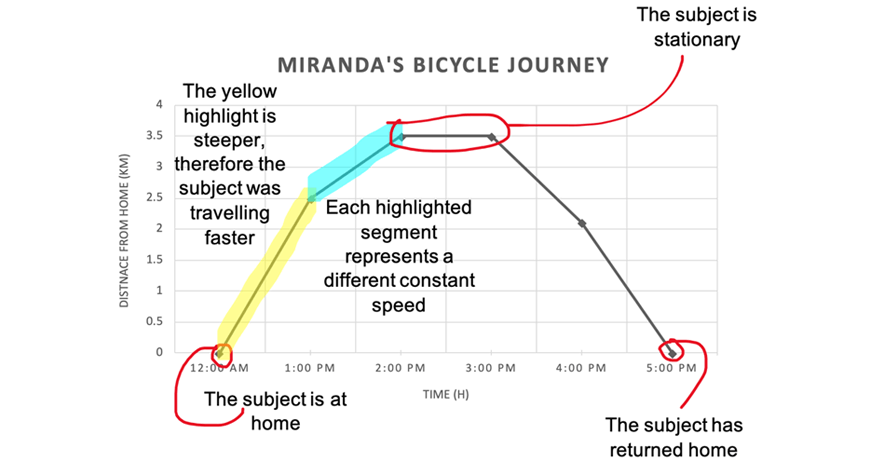### Example

The graph below shows the journey of two different people, A & B.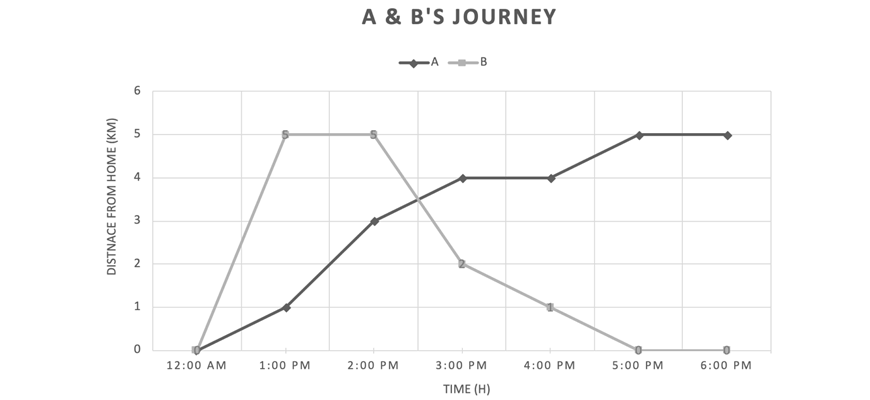Determine:

1. When was Person A was stationary?
2. When was Person B was stationary?
3. Person A’s fastest speed
4. Person B’s fastest speed
5. Did A or B return home?

Solution:

1. When was Person A was stationary?

To determine when Person A was stationary, we must look for flat horizontal line segments in A’s journey.

From the graph above, we can see that there are flat horizontal lines from $$3:00 \ pm$$ to $$4:00 \ pm$$ and from $$5:00 \ pm$$ to $$6:00 \ pm$$.

Therefore, Person A was stationary from $$3:00 \ pm$$ to $$4:00 \ pm$$ and from $$5:00 \ pm$$ to $$6:00 \ pm$$.

2. When was Person B was stationary?

To determine when Person B was stationary, we must look for flat horizontal line segments in B’s journey.

From the graph above, we can see that there are flat horizontal lines from $$1:00 \ pm$$ to $$2:00 \ pm$$ and from $$5:00 \ pm$$ to $$6:00 \ pm$$.

Therefore, Person B was stationary from $$1:00 \ pm$$ to $$2:00 \ pm$$ and from $$5:00 \ pm$$ to $$6:00 \ pm$$.

3. Person A’s fastest speed

To determine Person A’s fastest speed, we must look for the steepest straight line in A’s journey.

From the graph above, we can see that the steepest straight line segment is from $$1:00 \ pm$$ to $$2:00 \ pm$$.

Since $$gradient \ = \ speed$$, we must determine the gradient of this interval:

\begin{align*}
&= \frac{3.0 – 1.0}{1} \\
&= 2 \ km/h \\
&= Speed
\end{align*}

Therefore, Person A’s fastest speed was $$2 \ km/h$$

4. Person B’s fastest speed

To determine Person B’s fastest speed, we must look for the steepest straight line in B’s journey.

From the graph above, we can see that the steepest straight line segment is from $$12:00 \ pm$$ to $$1:00 \ pm$$.

Since $$gradient \ = \ speed$$, we must determine the gradient of this interval:

\begin{align*}
&= \frac{5.0 – 0.0}{1} \\
&= 5 \ km/h \\
&= Speed
\end{align*}

Therefore, Person B’s fastest speed was $$5 \ km/h$$

5. Did A or B return home at the end of the journey?

Person A or B would have returned home if there distance from home was $$0 \ km$$ at the end of the graph.

From observing the graph, it is clear that only Person B had a distance of $$0 \ km$$ from home at $$6:00 \ pm$$.

Therefore, only Person B returned home at the end of the journey.

### Common mistakes

Common mistakes students make with travel graphs are:

• Not being able to correctly identify steeper gradients.

## Checkpoint questions

1. It takes Tim and Taylor $$24 \ hours$$ to paint $$8 \ fences$$. How long will it take them to paint $$17 \ fences$$?

2. Samantha bought $$5 \ kg$$ of apples for $$24.50$$ at Woolworths, $$3 \ kg$$ of apples for $$23.42$$ at Coles and $$4 \ kg$$ of apples for $$22.10$$ at the local supermarket.

Determine which purchase was the most value and how much it would have cost to buy $$12 \ kg$$ of apples from that place.

3. A recipe which reserves $$4$$ people requires $$770 g$$ of chicken. If I want to feed $$7$$ people using this recipe, how much chicken will I have leftover if I buy $$2 kg$$?

4. Steve can walk $$1300 \ metres$$ in $$35 \ minutes$$. How long will it take him to walk a $$6 \ km$$ marathon?

5. Miranda walked at a speed of $$3 \ km/h$$ for $$23 \ minutes$$ and then drove a car at a speed of $$70 \ km/h$$ for $$10 \ minutes$$. How far did she travel?

6. A train travelled $$40 km/h$$ for $$10 minutes$$ and then $$80 km/h$$ for $$14 minutes$$. What was the average speed of the train?

7. A human body requires $$0.32 \ mg/L$$ of iron every $$24 \ hours$$. Express this rate in $$kg/mL$$.

8. A plane is travelling at $$30 \ m/s$$. Convert its speed into kilometres per day.

9. Steph had a heart rate of $$178 \ bpm$$. How many beats would his heartbeat per day?

10. Alicia rode her bike from her house to the local train station. There she waited $$1 \ \text{hour}$$ before boarding a train.

However, the train broke down halfway and Alicia was forced to walk the rest of the way to her destination.

After meeting with some friends, she rode a bike caught an Uber, walked back home, but in no particular order.

Using the graph below, determine Alicia’s mode of transport for each straight line segment.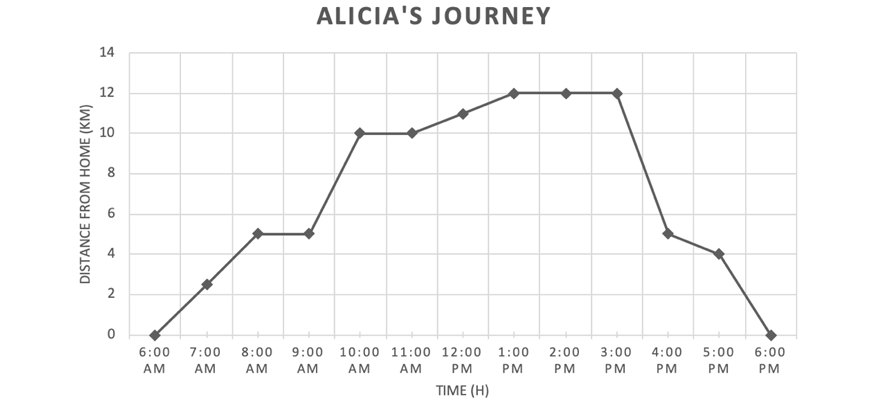## Solutions

1.

\begin{align*}
24 \ hours \ &/ \ fence \\
3 \ hours \ &/ \ 1 \ fence
\ ( \text{Divided  by} \ 8 ) \\
51 \ hours \ &/ \ 17 \ fence
\ (\text{Multiplied by} \ 17)
\end{align*}

Therefore, it will take them $$51 \ hours$$ to paint $$17 \ fences$$.

2.

\begin{align*}
$24.50 \ &/ \ 5kg \ (W) \\$4.90 \ &/ \ 1kg
\ (\text{Divided by} \ 5)
\end{align*}

\begin{align*}
$23.42 \ &/ \ 3kg \ (C) \\$7.81 \ &/ \ 1kg
\ (\text{ Divided by} \ 3)
\end{align*}

\begin{align*}
$22.10 \ &/ \ 4kg \ (LS) \\$5.53 \ &/ \ 1kg
\ (\text{Divided by} 4)
\end{align*}

Therefore, Woolworths is the most value.

To find out how much it would cost to buy $$12 \ kg$$ of apples from Woolworths, multiply the simplified rate by $$12$$:

\begin{align*}
\$58.80 \ \text{for} \ 12 \ kg \ \text{of apples from Woolworths}
\end{align*}

3.

\begin{align*}
770 \ g \ &/ \ 4 \ people \\
192.5 \ g \ &/ \ 1 \ person
\ (\text{Divided by} 4) \\
1347.5 \ g \ &/ \ 7 \ people
\ (\text{Multiplied by} 7)
\end{align*}

Therefore, remainder form $$2kg$$:

\begin{align*}
2000 \ – \ 1347.5 \ = \ 652.5 \ g
\end{align*}

Hence, $$652.5 \ g$$ of chicken will be left over.

4.

$$1300 \ m \ / \ 35 \ minutes$$

\begin{align*}
Time &= \frac{Distance}{Speed} \\
&= \frac{6000}{ \frac{1300}{35}} \\
&= 161.54 \ minutes
\end{align*}

Therefore, it will take Steve $$161.54 \ minutes$$.

5.

Using the formula:

\begin{align*}
Distance \ &= \ Speed \times Times \\
&= 3 \times \frac{23}{60} + 70 \times \frac{10}{60} \\
&= 12.82 \ km
\end{align*}

Hence, Miranda walked a total of $$12.82 \ km$$.

6.

Since $$Average \ Speed \ = \ Total \ Distance$$ divided by $$Total \ Time$$, we must first figure out the total distance.

Using the formula:

\begin{align*}
Total \ distance \ &= \ Speed \times \ Time \\
&= 40 \times \ \frac{10}{60} + 80 \times \frac{14}{60} \\
&= 25.22 \ km \\
\end{align*}

\begin{align*}
Total \ Time &= \ 10 \ mins + 14 \ mins \\
&= 21 \ minutes \\
\end{align*}

\begin{align*}
Avg. Speed &= \frac{Total \ Distance}{Total \ Time} \\
&= \frac{12.33}{\frac{24}{60}} \\
&= 30.83 \ km/h
\end{align*}

7.

First convert $$0.32 \ mg/L$$ into $$x \ mg/mL$$.

To do this, divide by $$1000$$:

\begin{align*}
0.00032 \ mg/ml
\end{align*}

Then convert into $$y \ kg/L$$.

To do this, divide by $$1000$$:

\begin{align*}
0.00000032 \ mg/ml
\end{align*}

8.

First convert $$30 \ m/s$$ into $$x \ m/day$$.

To do this, multiply by $$8640$$:

\begin{align*}
259 \ 200 \ m \ / \ day
\end{align*}

Then convert into $$y \ km \ / \ day$$.

To do this, divide by $$1000$$:

\begin{align*}
259.2km \ / \ day
\end{align*}

9.

To answer this question, we must convert $$178 \ bpm$$ into $$x \ beats$$.

To do this, multiply by $$1440$$:

\begin{align*}
256 \ 320 \ beats \ / \ day
\end{align*}

10.

As the question states, the first straight line interval $$[6:00 \ am \ to \ 8:00 \ am]$$ must be through bike.

She was then stationary for the next flat horizontal line interval $$[8:00 \ am \ to \ 9:00 \ am]$$.

The next straight line interval must then represent the train journey $$[9:00 \ am \ to \ 10:00 \ am]$$.

The following flat horizontal line interval represents a stationary train after it broke down $$[10:00 \ am \ to \ 11:00 \ am]$$.

Therefore, as the question states, the next straight line interval must represent walking $$[11:00 \ am \ to \ 1:00 \ pm]$$.

After her meeting with friends where she is stationary $$[1:00 \ pm \ to \ 3:00 \ pm]$$, we are given $$3$$ different straight line intervals and $$3$$ different modes of transport (car, bike walk).

The car is clearly the fastest mode of transport and must be represented by the straight line interval with the steepest gradient $$[3:00 \ pm \ to \ 4:00 \ pm]$$.

Similarly, walking is the slowest mode of transport and must be represented by the straight line interval with the shallowest gradient $$[4:00 \ pm \ to \ 5:00 \ pm]$$.

This leaves the bike as the mode of transport for the last interval $$[5:00 \ pm \ to \ 6:00 \ pm]$$.

## Summary of Rates and Ratios

Questions involving rates are fundamental part of junior mathematics.

It is important to develop a good foundation in this area as this is a concept which is heavily present in harder senior topics.

Always pay attention to the techniques you can apply when approaching a question as well as the variety of common mistakes you should watch out for.

Finally, we hope that you’ve learnt something new from this subject guide, so get out there and ace mathematics!

© Matrix Education and www.matrix.edu.au, 2021. Unauthorised use and/or duplication of this material without express and written permission from this site’s author and/or owner is strictly prohibited. Excerpts and links may be used, provided that full and clear credit is given to Matrix Education and www.matrix.edu.au with appropriate and specific direction to the original content.